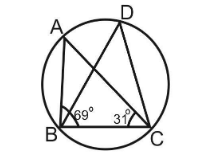In figure ∠ABC = 69∘, ∠ACB= 31∘, find ∠BDC.

# In figure ∠ABC = ${69}^{\circ }$, ∠ACB= ${31}^{\circ }$, find ∠BDC.1. A
${38}^{\circ }$
2. B
${80}^{\circ }$
3. C
${100}^{\circ }$
4. D
None of these

Fill Out the Form for Expert Academic Guidance!l

+91

Live ClassesBooksTest SeriesSelf Learning

Verify OTP Code (required)

### Solution:

By angle sum property of triangle we have
BAC + ∠ABC + ∠ACB = ${180}^{\circ }$
$⇒$ BAC + ${69}^{\circ }$+ ${31}^{\circ }$= ${180}^{\circ }$
⇒ ∠BAC = ${80}^{\circ }$
BAC and BDC are angles subtended by chord BC on the arc of a circle.
⇒ ∠BDC = ${80}^{\circ }$
So, Option 2 is correct.

## Related content

 Area of Square Area of Isosceles Triangle Pythagoras Theorem Triangle Formula Perimeter of Triangle Formula Area Formulae Volume of Cone Formula Matrices and Determinants_mathematics Critical Points Solved Examples Type of relations_mathematics+91

Live ClassesBooksTest SeriesSelf Learning

Verify OTP Code (required)